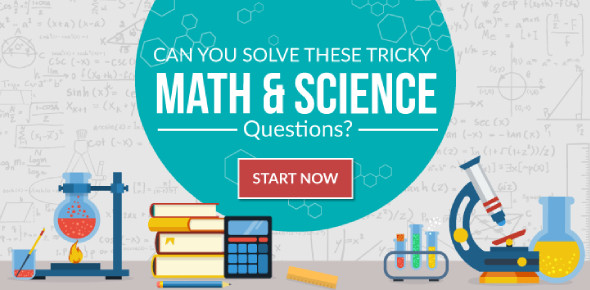# Math N' Science Quiz

12 Questions | Total Attempts: 7301SettingsMath and science have been closely related and science depends a lot more on some math. Some of the brightest students in the schools have perfect knowledge of math and science. If you consider yourself to be in that class of students then the test below is perfect to test how true that notion is. Try it out and share your results!

• 1.
Using 150 characters, describe your favorite operation in Math
• 2.
• A.

the middle of the diameter of a circle formula

• B.

A word of course duh

• C.

The circumference of a circle formula

• D.

Dont know, I am only gr. 1-6

• 3.
What is the key word of a gold element?
• A.

At

• B.

As

• C.

Au

• D.

H

• E.

O

• 4.
What is a nucleus?
• A.

A....ahhh...what is it?

• B.

The function of an atom

• C.

Algebra

• D.

A group of protons and neutrons joined by a strong force

• E.

A group of area formulas

• 5.
What is the limit of a constant over a variable as the variable approaches zero?
• A.

Zero

• B.

Infinity

• C.

3.14

• D.

The unknown

• 6.
What is the diameter of a cricle if the raidus is 30?
• 7.
What is the formula of rectangle?
• A.

Lw

• B.

A+b+c

• C.

S X s

• D.

Pi r squared

• 8.
What is the limit of a function as X aproaches A, if A is equal to D, what happens if X mixes both symbols and minus the X to mixed A and D?
• A.

I dont like Geometry!

• B.

Value of 23 squared

• C.

Pi r sub 1 r sub 2

• D.

SYNTAX ERROR

• E.

Uh...um...is it infinity?

• 9.
What is a number
• A.

I think I cant answer that

• B.

A number will be always a number :)

• C.

Well U see a number is like...ah... example, 1 is pronounced as one, you know numbers, you are just fooling us >:(

• D.

1,2,3,4,5,6,7,8,9 and 10 YAHOO!!!!!

• E.

You are a number! :D

• 10.
Answer only Yes or No - Are you a psychic?
• 11.
This is the last question
• A.

Ya! yaaaah!

• B.

I dont want to

• 12.
What is 7346734683764037 X 46783463746387
• A.

I bet you dont know the answer either huh?

• B.

6327153962736247964542345315621532525825821518572444759

• C.

I think the equation's wrong

• D.

I quit! I am not smart at math! now leave me alone!

Related TopicsBack to top